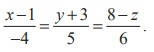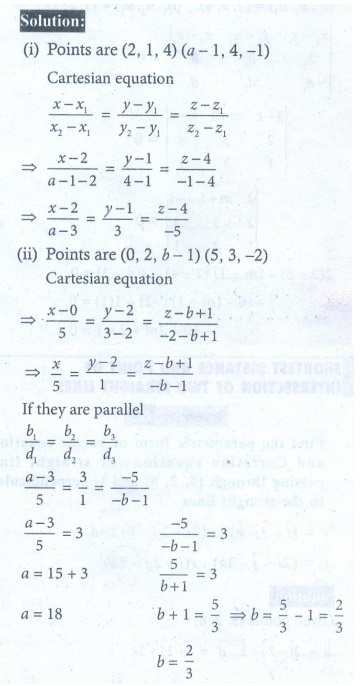Home | | Maths 12th Std | Exercise 6.4: Application of Vectors to 3-Dimensional Geometry

# Exercise 6.4: Application of Vectors to 3-Dimensional Geometry

Maths Book back answers and solution for Exercise questions - Mathematics : Application of Vectors to 3-Dimensional Geometry: Questions with Answer

EXERCISE 6.4

1. Find the non-parametric form of vector equation and Cartesian equations of the straight line passing through the point with position vector 4iˆ + 3 ˆ- 7kˆ and parallel to the vector 2iˆ - 6 ˆ+ 7kˆ .2. Find the parametric form of vector equation and Cartesian equations of the straight line passing through the point (-2, 3, 4) and parallel to the straight line3. Find the points where the straight line passes through (6, 7, 4) and (8, 4, 9) cuts the xz and yz planes.4. Find the direction cosines of the straight line passing through the points (5, 6, 7) and (7, 9,13) . Also, find the parametric form of vector equation and Cartesian equations of the straight line passing through two given points.5. Find the acute angle between the following lines.(iii) 2= 3= -and 6= - = -4z6. The vertices of ΔABC are A(7, 2,1), B(6, 0, 3) , and C(4, 2, 4) . Find ABC7. If the straight line joining the points (2,1, 4) and (a -1, 4, -1) is parallel to the line joining the points (0, 2, -1) and (5, 3, -2) , find the values of and b .8. If the straight linesare perpendicular to each other, find the value of .9. Show that the points (2, 3, 4),(-1, 4, 5) and (8,1, 2) are collinear.